# SSAT Upper Level Math : How to find the probability of an outcome

## Example Questions

### Example Question #51 : Probability

In a particular high school, 200 students are freshmen, 150 students are sophomores, 250 students are juniors, and 100 students are seniors. Twenty percent of freshmen are in honors classes, ten percent of sophomores are in honors classes, twelve percent of juniors are in honors classes, and thirty percent of seniors are in honors classes.

If a student is chosen at random, what is the probability that that student will be a senior student and a student who does not attend honors classes?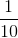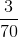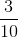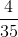Explanation:

First calculate the number of students: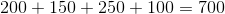The percentage of seniors that do not attend honors classes is: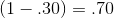Therefore, the probability of selecting a student who is a senior and one who does not attend honors classes is: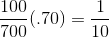### Example Question #21 : Probability

Toss a coin twice.  Roll a six sided dice once.  What is the probability of flipping two heads and rolling a one?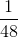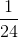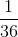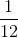Explanation:

Each toss and roll of the dice are independent events.  The probability of rolling either a head or tail on the first and second trial is one-half.

The probability of rolling any number on the dice issince there is one number on each of the six faces of the dice.

Multiply all the probabilities.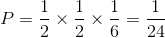### Example Question #41 : Data Analysis / Probablility

A standard deck of 53 cards (including the joker) is altered by adding the joker and the aces from another deck. What are the odds against a randomly drawn card being an ace?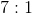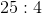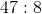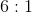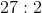Explanation:

Adding the joker and the four aces to a 53-card deck will yield a deck of 58 cards, which contains 8 aces and 50 other cards. The odds against drawing an ace at random are therefore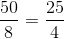- that is, 25 to 4.

### Example Question #22 : Outcomes

A standard deck of 52 cards is altered by adding the hearts from another deck. What are the odds against a randomly drawn card being a jack?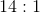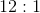None of the other responses give the correct odds.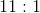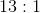Explanation:

Before the addition of the hearts, the deck had 4 jacks and 48 other cards. The thirteen hearts from the other deck include 1 jack and 12 other cards, so the altered deck contains 5 jacks and 60 other cards. That makes the odds against drawing a jack at random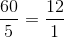- that is, 12 to 1, just as in the unaltered deck.

### Example Question #51 : Data Analysis / Probablility

A standard deck of 52 cards is altered by adding the jacks from another deck. What are the odds against a randomly drawn card being a club?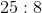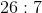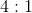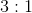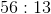Explanation:

Before the addition of the jacks, the deck had 13 clubs and 39 other cards. The 4 jacks from the other deck include 1 club and 3 other cards, so the altered deck contains 14 jacks and 42 other cards. That makes the odds against drawing a club at random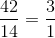- that is, 3 to 1, just as in the unaltered deck

### Example Question #51 : Data Analysis / Probablility

Which of the following ways can you alter a standard deck of 52 cards in order to neither increase nor decrease the probability that a randomly-drawn card would be a king?

Remove both of the black queens.

Remove the jacks.

Add the clubs from another deck.

Add the aces from another deck.

Add the clubs from another deck.

Explanation:

In an unaltered deck, 4 of the 52 cards, or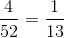of the cards, are kings. We are looking for an action that preserves this ratio.

We see that if the clubs from another deck are added, then there are 5 kings (the 4 original kings plus an added king) and 65 cards total (the original 53 plus the 13 added clubs). 5 of the 65 cards, or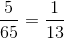of the cards, are kings, thereby preserving the ratio.

Of the other choices, none of them change the number of kings in the deck, but all change the total number of cards. Therefore, the ratio of kings to total cards must change.

### Example Question #1681 : Ssat Upper Level Quantitative (Math)

A standard deck of 53 cards (including the joker) is altered by adding the sevens from another deck. What are the odds against a randomly drawn card being a heart?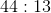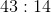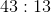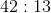Explanation:

Adding the four sevens from another deck to a 53-card deck will yield a 57-card deck with 14 hearts - the original 13 hearts and the added seven of hearts - and 43 other cards. Therefore, the odds against drawing a heart will be 43 to 14.

### Example Question #52 : Data Analysis / Probablility

Which of the following ways can you alter a standard deck of 52 cards in order to neither increase nor decrease the probability that a randomly-drawn card would be a spade?

None of the other responses gives a correct answer.

Remove the red fours and the black fives.

Add the red sevens from another deck.

Add the clubs from another deck.

Remove the red fours and the black fives.

Explanation:

In an unaltered deck, 13 of the 52 cards, or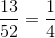of the cards, are spades. We are looking for an action that preserves this ratio.

If the red fours and the black fives, including the five of spades, are removed, then there are 12 spades left out of 48 cards, which means that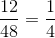of the cards are spades. This is the correct choice.

Of the other choices, none of them change the number of spades in the deck, but all change the total number of cards. Therefore, the ratio of spades to total cards must change.

### Example Question #86 : Probability

Aaron, Gary, Craig, and Boone are sitting down in a row of four chairs. What is the probability that Aaron and Gary will be seated beside each other?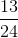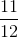Explanation:

Consider first all of the possible ways the men may be arranged, which is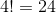Now, consider all of the ways that Aaron and Gary could be seated beside each other; it may be easier to visualize by drawing it out:

1. A G _ _
2. G A _ _
3. _ A G _
4. _ G A _
5. _ _ A G
6. _ _ G A

As seen, there are six possibilities.

Finally, for each of these cases, Craige and Boone could be seated in one of two ways.

So the probability that Aaron and Gary will be seated beside each other is: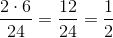### Example Question #52 : Data Analysis / Probablility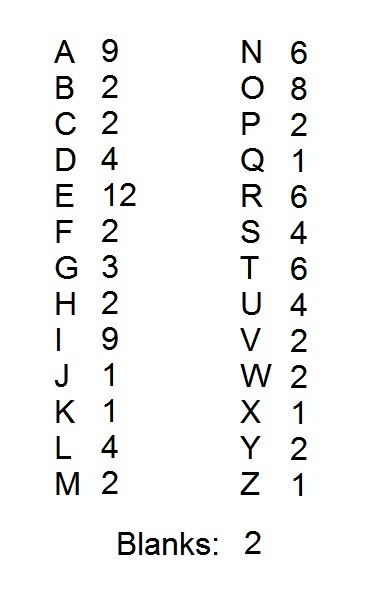A popular word game uses one hundred tiles, each of which is marked with a letter or a blank. The distribution of the tiles is shown above, with each letter paired with the number of tiles marked with that letter. Notice that there are two blank tiles.

All of the tiles are placed in a large box. What is the probability that a randomly drawn tile will be a vowel?

Note: for purposes of this question, "Y" is a consonant.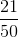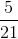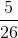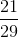Explanation:

Out of the 100 tiles, there are nine "A" tiles, twelve "E" tiles, nine "I" tiles, eight "O" tiles, and four "U" tiles. This is a total of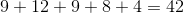tiles out of 100.

This makes the probability of drawing one of these tiles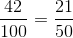### All SSAT Upper Level Math Resources